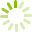# Probability and Statistics for Science and Engineering (MATH 2700)

This course explores the mathematical theory of probability and statistics and is intended for students in Science, Engineering, Computer Science and Business degree programs. Students are introduced to the concepts of descriptive statistics, laws of probability, probability distributions for discrete, continuous and jointly distributed random variables, laws of expectation, estimation, hypothesis testing, correlation and regression.
Course code: MATH 2700
Credits: 3.0
Length: 60.0 hours
Course outline: view https://www.vcc.ca/vccphp/courseoutline?subject=MATH&number=2700

## Prerequisites

MATH 1200 with a C- or equivalent.